### Exploratory analysis of data for geostatistics: the QQ-plot

Following the article   Introduction to exploratory data analysis for geostatistics   we   will discuss each of the available tools to carry out the exploratory analysis of spatial data. We have already discussed  the histograms , and now we will address the QQ-Plots.

QQ-Plots (or Quantile-Quantile Diagrams) are graphs in which the quantiles of two distributions are plotted against each other.

Building a normal QQ-Plot

QQ-Normal Plot is the diagram that makes it possible to compare the distribution of the data of a batch with the so-called normal   or   Gaussian distribution. Here is an example.How to build it?AT –   The batch of data to be processed is ordered by value, from the smallest to the largest, and then the percentage of lower values ​​is calculated for each value. We plot the values ​​of the batch on the abscissa and the percentages on the ordinates. In this example on the ordinates for the value 2 corresponds 21% (0,21) of lower values ​​present in the batch (and thus 79% of values ​​greater than 2).

B-   The Gaussian function is plotted with the standard deviations on the abscissa and the frequency percentage inferior to this value on the ordinates. For a frequency equal to 21% (0.21), the standard deviation equals -0.85.

C- We create the QQ-Plot:

• we use the value (DV) for each data,
• we look for the percentage of graph A,
• with this percentage, we move to   graph B and obtain the corresponding standard deviation value (NV),
• we draw the point using NV on the abscissa and DV on the ordinates.

The right portion of the QQ-Plot indicates the position that should have the points if they matched exactly the normal distribution.

How to build a general QQPlot

The   QQ-General Plot   is used to evaluate the similarity between the distributions of two sets of data.Here we have two variables: Depth and Distance

How to build it?A –   As for the normal QQ-Plot, the first   batch of data to be processed is ordered by value, from the smallest to the largest, then the percentage of lower values ​​is calculated for each value. We plot on the ordinates the values ​​of the lot and on the abscissa the percentages. In this example for the value 2 in our data we have 21% (0,21) of lower values ​​present in the batch.

B –   The second batch of data is treated in the same way. In this example for the value in our data we have 37% (0.37) of lower values ​​present in the batch. You will notice that there is no value in the batch with a frequency of 0.21 as in the first batch of data.

C- We create the QQ-Plot:

• for each data of lot A we select its value (DV1),
• we look for the percentage of graph A,
• with this percentage, we move to   graph B and obtain the value of the corresponding batch B (DV2), either by selecting it directly (whenever possible), or by interpolating between the two encompassing values ​​, as in the example above.
• we draw the point using DV2 on the abscissa and DV1 on the ordinate,
• for each data of the batch B we select its value (DV2),
• we look for the percentage of chart B,
• using this percentage, we move to   graph A and obtain the value of the corresponding batch A (DV1), either by selecting it directly (whenever possible), or by interpolating between the two values ​​which encompass it, as in the example above.
• we draw the point using DV2 on the abscissa and DV1 on the ordinate.

Unlike the normal QQ-Plot, we can not draw theoretical line because we do not know the distribution function of the lots A and B. However, if the two distributions are exactly the same, the points will be aligned on a straight line. In the above example (Depth-Distance) this is not the case.
The following example is a perfect match (since it’s the same variable):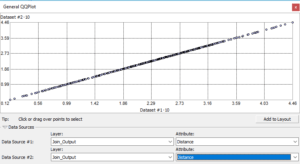Interpretation of QQ-Plots

We will repeat here what we have, already, said for the histograms:

Some kriging methods work best if the data is distributed approximately normally (the bell-shaped curve).
In particular, quantile and probability maps using ordinary, simple and universal kriging assume that the data come from a normal distribution.
As we saw in the previous article, kriging is also based on the hypothesis of stationarity.   This assumption requires, in part, that all data values ​​come from distributions that have the same variability. In nature, we often observe that as values ​​increase, their variability, also, increases.   The   transformations of source data can be used to transform your data into a normal  distribution and satisfy the assumption of equal variability for the whole set.

Therefore, we will look for the same things as with the histograms, but with the QQ-Plot it will be easier.

If we select the variable   Depth, used for the histogram and we draw its normal QQ-Plot we have: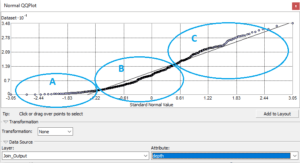We have three different areas:

• A- Points to the left of the theoretical line, very far from this one
• B- Points to the right of the theoretical line, and
• C- Points to the left, again

The general shape is equivalent to an S.

The information that we can draw from the general shape of the points curve is mainly related to the form coefficients: skewness and kurtosis. Moreover, we can immediately observe if our data follow a mono or bi-modal curve.

First, a few words on spreading ( skewness ).

We have three main types of distribution: normal, moved to the left (towards the small values ​​of our data), moved to the right (towards the big values ​​of our data).

In order to, quickly, find which the type of our distribution is; look at the corresponding QQ-Plot area in the centre of our distribution (Value 0 of the standard deviation):

UNBIASED DISTRIBUTION (NORMAL):The points of the data corresponding to the centre of the distribution are included (or very close) in the theoretical line.

DISTRIBUTION BIASED to the LEFT: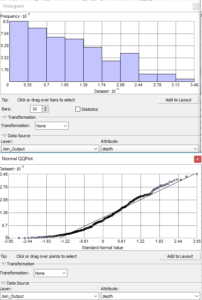The area of ​​points around 0 standard deviation is substantially below the theoretical line.

DISTRIBUTION BIASED TO THE RIGHT: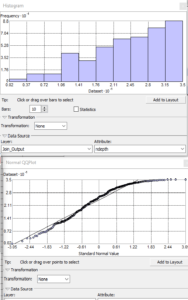The area of ​​points around 0 standard deviation is substantially above the theoretical line.

Observation of the flattening

The other possible observation concerns the coefficient of spread (kurtosis).

KURTOSIS LESS THAN 3

Distributions with relatively thin edges (called platykurtic) and which have a kurtosis value lower than 3, have a general S shape, with the negative part of the standard deviations concave, and the positive part convex:KURTOSIS GREATER THAN 3

Distributions with relatively thick edges (called leptokurtic)   and which have a kurtosis  value greater than 3, have a general inverted S shape, with the negative section of the standard deviations convex, and the positive section concave: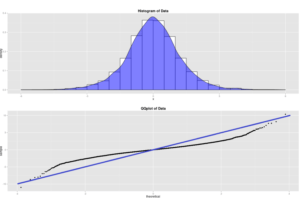What to do?

Using the normal QQ-plot there are two things we can do: find a transformation that brings our data back to a normal (or near) distribution and identify the data that can be problematic.

If we consider the first diagram of this article, it is easier to find the exponential transformation (Box-Cox) with the QQ-Plot than with the histogram:When we modify the transformation parameter, we get a better picture of the adequacy to the theoretical line..

The other interesting aspect of the Geostatistical Analyst is the link between the diagram tools, here the QQ-plot and the display in ArcMap. If you use the selection tool on QQ-Plot points you will observe the selected points on the map.

If you select points that deviate from the normal line for large values:We realize that they are all in the periphery of the area considered. They can therefore translate an external phenomenon to our zone. This will be kept in mind, for example, to test the quality of the final interpolation with or without these points.

If we do the same thing for points deviating in small values: figura 14

We observe that the distribution of these points belong to the phenomenon intrinsic to the area considered, or that, in any case, it will be necessary to take them into account for the modelling of the interpolation function.

In the next article we will see how to detect outliers with Voronoi polygons.